Human beings get acquainted with geometrical shapes ever since infancy. Patterns, designs and shapes are an integral part of our daily life as every aspect of our environment like curves, angles and lines form our aesthetic. Singular representations with definite properties define shapes. Some of the common geometrical shapes are polygons like circles, squares, quadrangles, rectangles or triangles, and parallelograms, trapezoids, rhombus, and solids (which include cylinders, pyramids, prisms.) among many others.

## Basic Geometrical Shapes

Geometrical shapes are mostly two or three dimensional in nature. In the following tables, we shall become acquainted with some of the most common 2D and 3D geometric shapes that come in use.

 Shape Description PolygonA closed figure with line segments which intersect with two other line segments Quadrilateral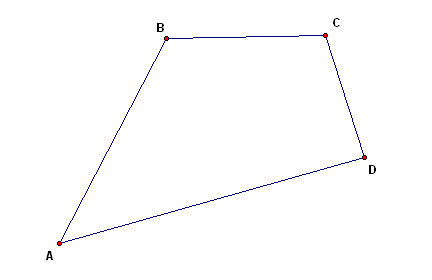A four-sided polygon is called a quadrilateral SquareA quadrilateral which has sides that are equal in length and form right angles TriangleA three-sided polygon the sum of whose internal angles are 180 degrees Rectangle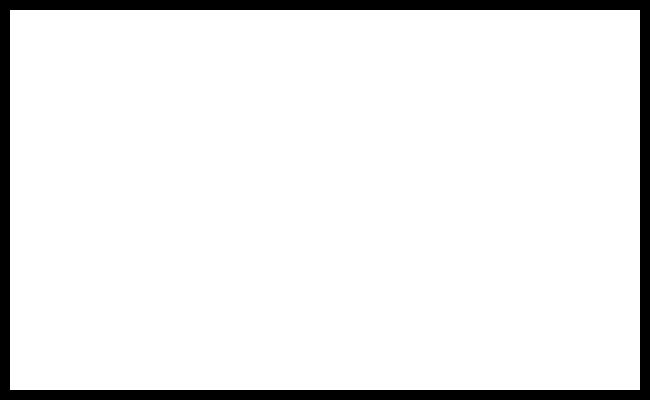A four-sided polygon all of whose sides form right angles Parallelogram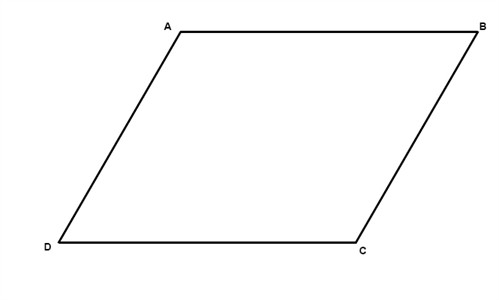Two pairs of parallel sides on a four-sided polygon Pentagon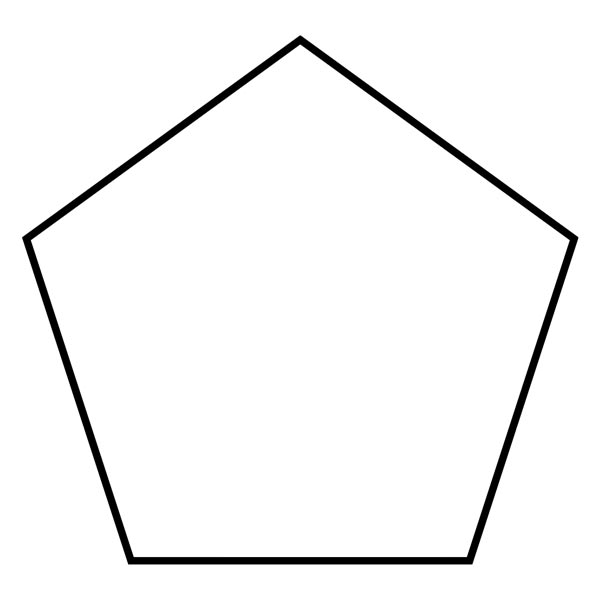A polygon that has five sides Hexagon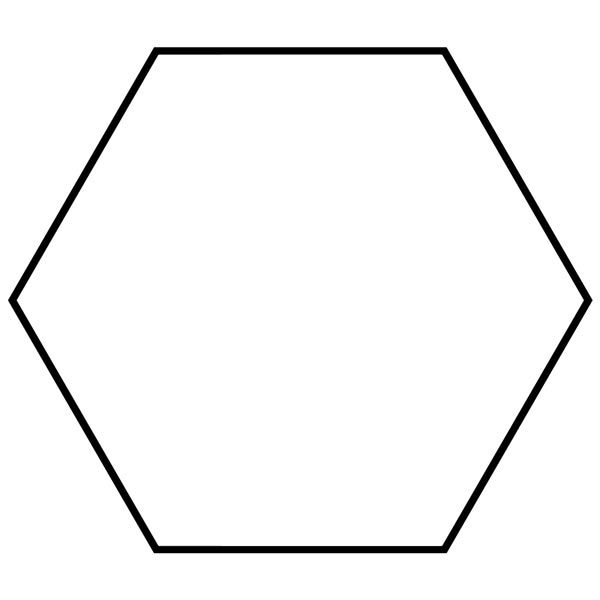A polygon that has six sides Heptagon A polygon that has seven sides Octagon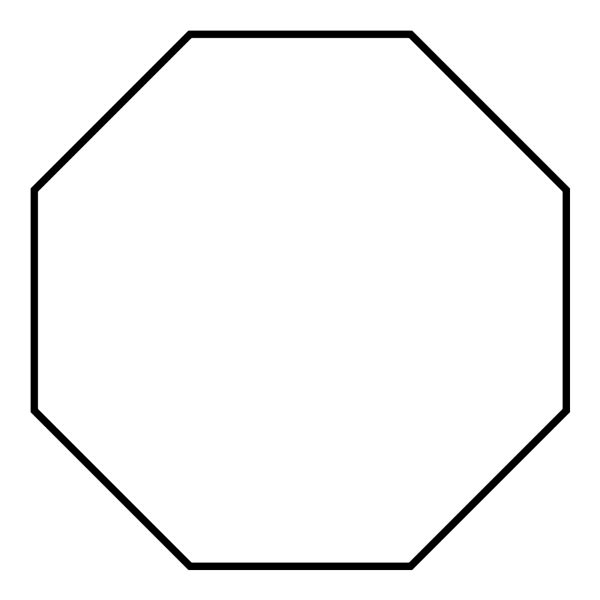A polygon that has eight sides

Table 1: Shows and describes some common kinds of 2D geometric shapes

Apart from the mentioned 2D geometric shapes described and illustrated in the table above, some other common, curved 2D geometric shapes would be:

1. Circles: The locus of all points measure the same from a singular central point. These generally have lines of symmetry that are infinite.
2. Ellipse: An ellipse has two similar points called focus the sum of whose distance remains the same from any point on the ellipse. These generally have two lines of symmetry.
3. Oval: This is a general term that can be used for ellipses as well. It stands for both regular and irregular curves shaped like eggs.
4. Arch: A curved path from one point of a circle to another, which can also be considered a part of its circumference.
5. Lens: These are circular arcs that meet with each other through their endpoints. It is generally a biconvex shape.
6. Annulus: When the centre of a disk is removed, the shape formed resembles two small rings, one engulfed in the other.Figure 1a illustrates a circle, an ellipse, a general oval shape as well as a stadium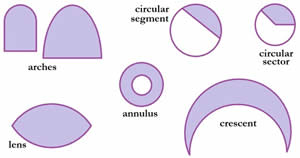Figure 1b illustrates arches, circular segments, annuluses, crescents, lenses and circular sectors

 Shapes Description PrismsA prism generally has two congruent and parallel faces. A cube is also a kind of prism, but it has six congruent faces. There are rectangular prisms, triangular prisms, hexagonal prisms. PyramidA pyramid or a polyhedron whose base is a polygon with all lateral faces that are mostly congruent in character. A polygon is more often than not described by the shape of the base it has. CylinderA cylinder has a flat base, a flat top and a curved side. The middle section usually represents a wrapped up rectangle. The difference between a prism and a cylinder is that a cylinder has a circle for a base and not a polygon. Cone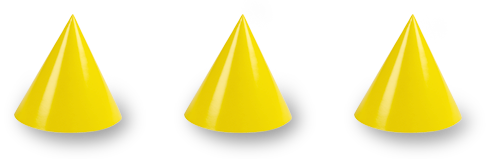A cone generally assumes a curved shape with a circular or oval base which gets narrower towards a point and it has a singular vertex. SphereA sphere generally has neither vertices nor edges whereas the distance from any point in the sphere to the centre always remains constant.

Table 2:  Shows and describes some common 3D geometric shapes

## List of relevant terms and their definitions

The following is a list of a few terms and the definition of the same that might be of use to the readers in the future:

1. Point: something that denotes a location or dot on space or paper
2. Line: something that connects two such dots only to continue forever in both directions
3. Perpendicular line segments: sections of lines that cross at an angle of 90 degrees
4. Parallel line segments: sections of lines that never cross each other at any point
5. Right angle: an angle whose measurement is 90 degrees
6. Acute angle: an angle whose measurement is less than 90 degrees
7. Obtuse angle: an angle whose measurement is more than 90 degrees
8. Radius: a line segment that helps identify the distance between the center of the circle to any point on the circumference of the circle
9. Diameter: it’s usually measured at twice the length of the radius
10. Circumference: the perimeter or the distance around the circle
11. Chord: a section of a line that joins two points on a curve of a circle.

## List of relevant formulas

The following is a list of common mathematical formulas that come in use for geometric shapes:

1. Area of Square = length2 (l x l)
2. Area of Rectangle = length x height
3. Area of a triangle = 1/2 x length x height
4. Circumference of a circle = 2 pi r (pi x diameter)
5. Area of rectangular Prism = length x height x depth
6. Volume of cylinder = area of base x height pi (d/2)2 x h
7. Volume of a cone = 1/3 x area of base x height 1/3 x pi(d/2)2 x h

If you want to know more about the definitions and formulas of other geometrical shapes, visit here.

## Are you slow and accurate? Or quick but careless?

Practice from a bank of 300,000+ questions , analyse your strengths & improvement opportunities.

+91
No thanks.

## Request a Free 60 minute counselling session at your home

Please enter a valid phone number
•7,829,648

Happy Students
•358,177,393

Questions Attempted
•3,028,498

Tests Taken
•3,020,367